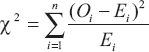# Cross Tabulation, Chi-Squared Test

Cross Tabulation is a main frame statistical model which follows on similar lines, it help you take informed decision with regards to your research by identifying patterns, trends and correlation between parameters within your study. When conducting a study, the raw data can usually be daunting and will always points to several chaotic possible outcomes, in such situation cross-tab helps you zero in on a single theory beyond doubt by drawing trends, comparisons and correlations between factors that are mutually inclusive within your study.

For example, consider your college application – you probably did not realize it at the time but you were mentally cross tabulating the factors involved to arrive at a conscious decision with respect to which colleges you wanted to attend and had the best shot at while applying. Let us go through your decision making process one factor at a time.

First, you needed to look at the academic factor which were your grades throughout high school, SAT scores, the field you wanted to major in and the application essay you would need to write. Second, comes the financial factor which will look at the tuition fees and possibilities of a scholarship. Last, but definitely not the least, would be the emotional factor which will consider your distance from home and how far are the universities your friends are considering so reunions would not be an issue. In other words, cross tabulating Academics + Finance + Emotions led you to a refined list of universities one of which is or soon will be your Alma Mater.

Cross tabulation also known as cross-tab or contingency table is a statistical tool that is used for categorical data. Categorical data involves values that are mutually exclusive to each other. Data is always collected in numbers, but numbers have no value unless they mean something. 4,7,9 are simply numerical unless until specified. For example, 4 apples, 7 bananas, and 9 kiwis.

Cross tabulation is usually used to examine the relationship within the data that is not evident. It is quite useful in market research studies and in surveys. A cross tab report shows the connection between two or more question asked in the survey.

Understanding Cross Tabulation with Example

Cross-tab is a popular choice for statistical data analysis. Since it is a reporting/ analyzing tool it can used with any level of data: ordinal or nominal, because it treats all data as nominal data (nominal data is not measured it is categorized).

Let’s say you can analyze the relation between two categorical variable like age and purchase of electronic gadgets.

There are two questions asked here:

(ii) What is the electronic gadget that you are likely to buy in the next 6 months?

 Age Laptop Phone Tablet Digital Camera 20-25 38% 29% 31% 12% 25-30 19% 15% 24% 17% 30-35 23% 19% 11% 27% 35-40 19% 12% 9% 30% Above 40 12% 17% 5% 31%

In this example you can see the distinctive connection between the age and the purchase of the electronic gadget. It is not surprising but certainly interesting to see the correlation between the two variables through the data collected.

In survey research crosstab allows to deep dive and analyze the prospective data, making it simpler to spot trends and opportunities without getting overwhelmed with all the data gathered from the responses.

### Chi-Squared Test

A chi-squared test, also written as χ2 test, is any statistical hypothesis test where the sampling distribution of the test statistic is a chi-squared distribution when the null hypothesis is true. Without other qualification, ‘chi-squared test’ often is used as short for Pearson’s chi-squared test. The chi-squared test is used to determine whether there is a significant difference between the expected frequencies and the observed frequencies in one or more categories.

In the standard applications of the test, the observations are classified into mutually exclusive classes, and there is some theory, or say null hypothesis, which gives the probability that any observation falls into the corresponding class. The purpose of the test is to evaluate how likely the observations that are made would be, assuming the null hypothesis is true.

Chi-squared tests are often constructed from a sum of squared errors, or through the sample variance. Test statistics that follow a chi-squared distribution arise from an assumption of independent normally distributed data, which is valid in many cases due to the central limit theorem. A chi-squared test can be used to attempt rejection of the null hypothesis that the data are independent.

How to Calculate a Chi-square Statistics?

The formula for calculating a Chi-square statistic is:Where,

O stands for the observed frequency,

E stands for the expected frequency.

Expected count is subtracted from the observed count to find the difference between the two. Then the square of the difference is calculated to get rid of the negative vales (as the squares of 2 and −2 are, of course, both 4). Then the square of the difference is divided by the expected count to normalize bigger and smaller values (because we don’t want to get bigger Chi-square values just because we are working on large data sets). The sigma sign in front of them denotes that we have, to sum up, these values calculated for each cell.

As an example, suppose we want to find out that whether there is an association between smoking and lung disease.

The null and alternative hypothesis will be:-

H 0 : There is no association between smoking and lung disease.

H 1 : There is an association between smoking and lung disease.

## 2 thoughts on “Cross Tabulation, Chi-Squared Test”

error: Content is protected !!